FACTORS Statement
FACTORS factor-selections </ NOPRINT> ;

The FACTORS statement specifies the factors of the plan and generates the plan. Taken together, the factor-selections specify the plan to be generated; more than one factor-selection request can be used in a FACTORS statement. The form of a factor-selection is

name = m <OF n> <selection-type> ;
where
name

is a valid SAS name. This gives the name of a factor in the design.is a positive integer that gives the number of values to be selected. Ifis specified, the value ofmust be less than or equal to.is a positive integer that gives the number of values to be selected from.

selection-type

specifies one of five methods for selectingvalues. Possible values are COMB, CYCLIC, ORDERED, PERM, and RANDOM. The CYCLIC selection-type has additional optional specifications that enable you to specify an initial block of numbers to be cyclically permuted and an increment used to permute the numbers. By default, the selection-type is RANDOM, unless you use the ORDERED option in the PROC PLAN statement. In this case, the default selection-type is ORDERED. For details, see the following section, Selection-Types; for examples, see the section Syntax Examples.

The following option can appear in the FACTORS statement after the slash:

NOPRINT

suppresses the display of the plan. This is particularly useful when you require only an output data set. Note that this option temporarily disables the Output Delivery System (ODS); see Chapter 20, Using the Output Delivery System, for more information.

### Selection-Types

PROC PLAN interprets selection-type as follows:

RANDOM

selects thelevels of the factor randomly without replacement from the integers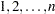. Or, ifis not specified, RANDOM selects levels by randomly ordering the integers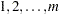.

ORDERED

selects the levels of the factor as the integers, in that order.

PERM

selects thelevels of the factor as a permutation of the integers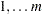according to an algorithm that cycles through all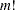permutations. The permutations are produced in a sorted standard order; see Example 67.6.

COMB

selects thelevels of the factor as a combination of the integers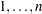takenat a time, according to an algorithm that cycles through all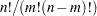combinations. The combinations are produced in a sorted standard order; see Example 67.6.

CYCLIC <(initial-block) > <increment>

selects the levels of the factor by cyclically permuting the integers. Wrapping occurs atifis not specified, and atifis specified. Additional optional specifications are as follows.

With the selection-type CYCLIC, you can optionally specify an initial-block and an increment. The initial-block must be specified within parentheses, and it specifies the block of numbers to permute. The first permutation is the block you specify, the second is the block permuted by 1 (or by the increment you specify), and so on. By default, the initial-block is the integers. If you specify an initial-block, it must havevalues. Values specified in the initial-block do not have to be given in increasing order.

The increment specifies the increment by which to permute the block of numbers. By default, the increment is 1.

### Syntax Examples

This section gives some simple syntax examples. For more complex examples and details on how to generate various designs, see Specifying Factor Structures. The examples in this section assume that you use the default random selection method and do not use the ORDERED option in the PROC PLAN statement.

The following specification generates a random permutation of the numbers 1, 2, 3, 4, and 5.

```factors A=5;
```

The following specification generates a random permutation of five of the integers from 1 to 8, selected without replacement.

```factors A=5 of 8;
```

Adding the ORDERED selection-type to the two previous specifications generates an ordered list of the integers 1 to 5. The following specification cyclically permutes the integers 1, 2, 3, and 4.

```factors A=4 cyclic;
```

Since this simple request generates only one permutation of the numbers, the procedure generates an ordered list of the integers 1 to 4. The following specification cyclically permutes the integers 5 to 8.

```factors A=4 of 8 cyclic (5 6 7 8);
```

In this case, since only one permutation is performed, the procedure generates an ordered list of the integers 5 to 8. The following specification produces an ordered list for A, with values 1 and 2.

```factors A=2 ordered B=4 of 8 cyclic (5 6 7 8) 2;
```

The associated factor levels for B are 5, 6, 7, 8 for level 1 of A, and 7, 8, 1, 2 for level 2 of A.

### Handling More Than One Factor-Selection

For cases with more than one factor-selection in the same FACTORS statement, PROC PLAN constructs the design as follows:

1. PROC PLAN first generates levels for the first factor-selection. These levels are permutations of integers (1, 2, and so on) appropriate for the selection type chosen. If you do not specify a selection type, PROC PLAN uses the default (RANDOM); if you specify the ORDERED option in the PROC PLAN statement, the procedure uses ORDERED as the default selection type.

2. For every integer generated for the first factor-selection, levels are generated for the second factor-selection. These levels are generated according to the specifications following the second equal sign.

3. This process is repeated until levels for all factor-selections have been generated.

The following statements give an example of generating a design with two random factors:

```proc plan;
factors One=4 Two=3;
run;
```

The procedure first generates a random permutation of the integers 1 to 4 and then, for each of these, generates a random permutation of the integers 1 to 3. You can think of factor Two as being nested within factor One, where the levels of factor One are to be randomly assigned to 4 units.

As another example, six random permutations of the numbers 1, 2, 3 can be generated by specifying the following statements:

```proc plan;
factors a=6 ordered b=3;
run;
```